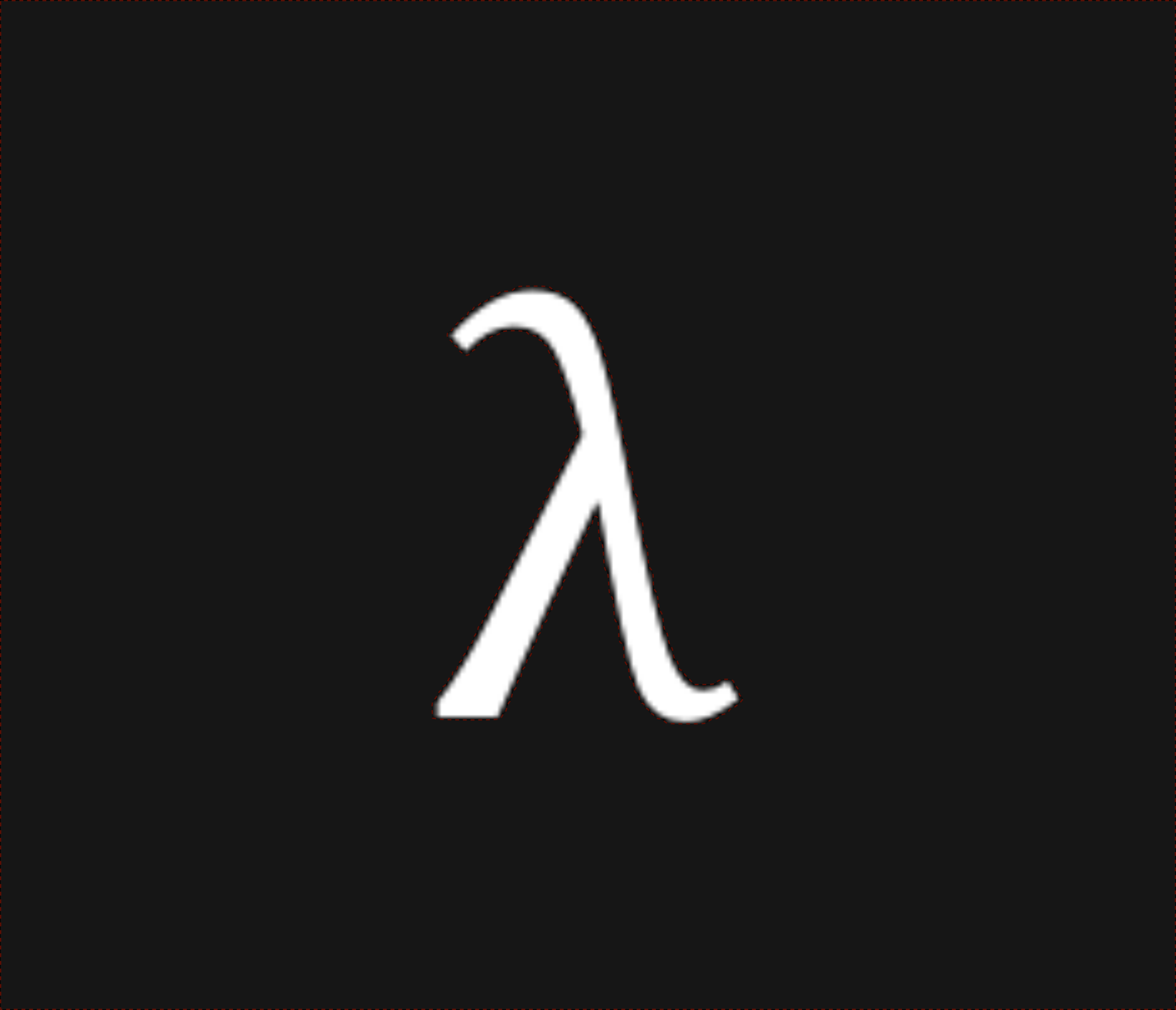nixpulvis

## The λ-calculus

λ-calculus one of the most fundamental programing languages, being made up of only three primative types of objects. It’s the basis for functional programming, and is just as powerful as any other programming language. I’ve created a parser and reducer for this language which, for example, will reduce (λx.x) 1 to 1. In addition to a parser, which consumes arbitrary text, a set of Rust macros: var!, abs! (λ!), and app! (γ!) are provided.

The λ-calculus is so very interesting to me, because like $$e^{\pi i + 1 = 0}$$, it is incredibly fundamental. Unlike Euler’s identity however, this calculus is at it’s core, actually very easy to understand.

All you have are three components:

• Names (e.g. x, or y)
• Abstractions (e.g. f(x) = x)
• Applications (e.g. f(g(x)))

For more detailed documentation of both this project, and the λ-calculus, read the source documentation. This page serves as a more personal account of my development, and both a (slightly) more approachable introduction to the λ-calculus, and some of the flexibility of Rust.

### A Brief History of λ

The λ-calculus was originally proposed as a tool for use in the construction of mathematics, however it’s be proven equivalent to all known programming languages . The practical implications of this are called the “Church-Turing thesis” or simply the computability thesis (complexity is another story).

I probably first heard about the λ back in grade school in some passing physics equation, almost certainly as wavelength. Despite how cool light is, it wasn’t until took my first class at Northeastern University and I learned about the function that I’ve started holding the λ above all.

TODO: Church Separates from State. TODO: λ as security parameter.

### Basic Syntax

There are a few grammars for the lambda calculus floating around out there, and we are also specifically aiming to support the simply typed λ-calculus, so we have our own parser (LALRPOP) with an unambiguous grammar to support this.

Below are some basic examples covering the different forms available in this grammar. Here the left hand side of -> parses into the right hand side.

x       -> x
x y     -> (x y)
(y x)   -> (y x)
λ       -> (λ_._)
λx      -> (λx._)
λx.x    -> (λx.x)
λx.(x)  -> (λx.x)
λx.x x  -> (λx.(x x))
(a b c) -> ((a b) c)


### Reductions

Here the left hand side of -> reduces to the right hand side. The beta reduction corresponds to calling a function to get it’s result. The simplest example is applying a single argument to the identity function, seen below:

(λx.x) y -> y


Functions are themselves values in the λ-calculus, making it what we call a higher order language, allowing us to write more complex reductions.

<- (λf.λg.λx.(f (g x))) (λx.x) (λx.x) y
-> (λg.λx.((λx.x) (g x))) (λx.x) y
-> (λx.((λx.x) ((λx.x) x))) y
-> (λx.((λx.x) x)) y
-> (λx.x) y
-> y

TODO: Visualize the multiple paths to y, reduction strategies.

(λx.λy.(y x)) first last -> last first


Even though all you seem to be able to do with this language is perform symbolic naming operations, it turns out that’s all you need to be able to do. In fact, with just this, you can write non-terminating reductions, for example the $$Ω$$ expression (called a combinator in this context):

Ω := (λx.x x) (λx.x x)
-> (λx.x x) (λx.x x)
-> (λx.x x) (λx.x x)
-> ...

• alpha
• beta
• eta

#### beta

###### Reduction Strategies
• Normal order
• Applicative Order
• Call by name
• Call by value

### Currying

Our grammar also supports currying, which allows us to write multi-argument functions on a language that fundamentally only allows one. This is done with the following scheme:

λx y -> λx.λy


This reads nicely, lambda x y is the same as lambda x, lambda y. To “call” a curried function simply call the result of each function call with the arguments in order.

(λx y.(add x y)) 1 2
-curry>
((λx.λy.(add x y)) 1) 2
-beta>
(λy.(add 1 y)) 2
-beta>


This works in any reasonable programming language with functions and arguments. In Ruby for example, you can write an add function like this if you’re so inclined.

def c_add(a)
lambda { |b| a + b }
end